# Uncharged capacitor connected to a charged capacitor

• Titan97

Gold Member

## Homework Statement

An uncharged capacitor is connected to a charged capacitor as shown: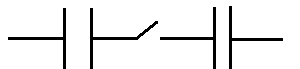The ends are left as it is.
Will the charges redistibute?

None

## The Attempt at a Solution

I think it won't.

Let the first capacitor be charged with charge Q. Before closing the switch, the charges are like: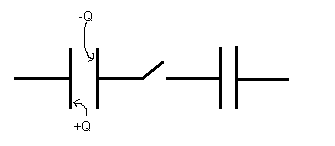If ##x## charge flows from the negative plate of first capacitor to the second capacitor, then a same ##x## has to flow from the first plate as well since opposite faces of a capacitor should have charges of same magnitude. So no charge transfer is possible.

This, however, is the reason given in my textbook:
" The positive charge on one face of capacitor will hold the negative charge in place. "

Is it correct? Because if I were to connect the two free ends to each other, then also no charge should redistribute. (I am also thinking about the problem 3.2 from Prucell and Morin).

See the case 1.

@gracy that's a totally different case. The wire does not form a loop.

This, however, is the reason given in my textbook:
" The positive charge on one face of capacitor will hold the negative charge in place. "

Is it correct? Because if I were to connect the two free ends to each other, then also no charge should redistribute. (I am also thinking about the problem 3.2 from Prucell and Morin).

The textbook is correct. Before the switch is closed the charges are held on the inside surfaces of the capacitor plates of the left-hand side capacitor by their mutual attraction. The leads of the charged capacitor will be at some potentials with respect to the zero potential reference at infinity. When the switch is closed there is no external potential difference added that can overcome this attraction. What does happen, however, is that the entire right hand capacitor assumes the potential of the first capacitor's right hand plate thanks to sharing a conductive path. Some very negligible charge displacement may occur in the conductors as a result, about the same as you would expect if the second capacitor were just a short length of wire instead of a capacitor.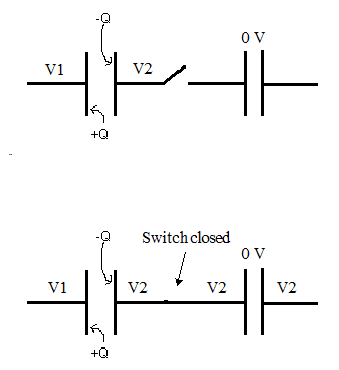If you close the loop by connecting the outer leads of the setup then a potential difference is established across the connecting wire and current will flow in that wire (very briefly if the wire's resistance is very small. Instantaneously if we're talking about an ideal conductor).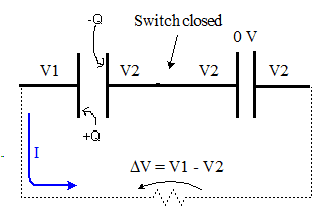Current will flow until this potential difference across the wire is eliminated, that is, when the potentials across the capacitors reach an equilibrium.

•cnh1995
@gneill is my reasoning correct?

@gneill is my reasoning correct?
Which reasoning are you referring to? The following bit?
Because if I were to connect the two free ends to each other, then also no charge should redistribute.
If so then no, because the potential difference established across the connecting wire will force charges to flow in that wire. When the charge moves, it can be pulled from the left hand plate of the left capacitor so long as an equal charge is pushed onto its right hand plate. The closed loop facilitates that.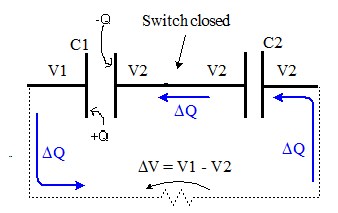I meant this:
If xxx charge flows from the negative plate of first capacitor to the second capacitor, then a same xxx has to flow from the first plate as well since opposite faces of a capacitor should have charges of same magnitude. So no charge transfer is possible.

Look at the direction of the conventional current flow that I drew in on the figure in post #6. The parcel of charge ΔQ moves from the positive plate of capacitor C1, through the wire, and onto the right plate of C2. In turn, this pushes the same amount of charge ΔQ off of the left plate of C2 and onto the right plate of C1 where it cancels the same amount of negative charge there. This all takes place in step, so that the charges on the plates of C1 remain equal and opposite. The same goes for the plates of C2.

•Titan97